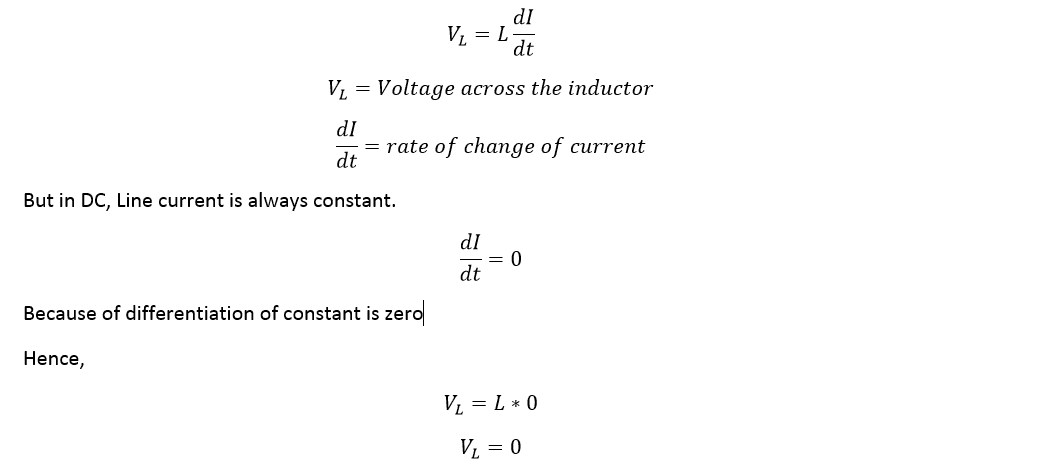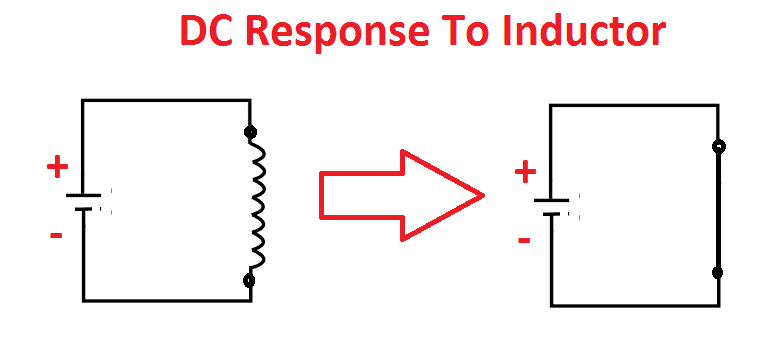# What happens if DC supply given to the Inductor

## What happens if DC supply given to the inductor:

Consider I is the current flow through an inductor circuit is DC, then the voltage V across the inductor isHence,

The Voltage across the inductor is zero, the voltage across any component is zero which means the resistance is zero, the inductor act as short circuit.

Also see: What are open circuit and its conditions.